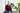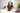15.6.2022 |

# Generators - what is this strange asterisk for?

A generator function is a special function whose body can be left at an arbitrary point and continued later on without losing its scope. It returns a `Generator` which in itself is both an iterator and iterable object. It offers a next, return and throw method. Inside a generator function a special `yield` keyword can be used to pass values out of the generator upon a call to `next()`. Generators yield values as long as they don't throw an error or return.

Examples:

``````describe('Generators', () => {
function* generateIdOnce(startId?: number) {
let lastId = startId ?? 0;
yield lastId++;
}

it('should generate a numerical id starting at 0 once', () => {
const generator = generateIdOnce();

expect(generator.next()).toEqual({ done: false, value: 0 });
expect(generator.next()).toEqual({ done: true, value: undefined });
});

function* generateInfiniteId(startId?: number): Generator {
let lastId = startId ?? 0;
while (true) {
yield lastId++;
}
}

const cases = new Array(99_999).fill(0).map((_, idx) => idx);
const infiniteIdGenerator = generateInfiniteId();

test.each(cases)('should generate a numerical id starting at 0 infinitely often', (expectedId) => {
expect(infiniteIdGenerator.next()).toEqual({ done: false, value: expectedId });
});

function* iterableGenerator() {
yield* new Array(5).fill(0).map((_, idx) => idx);
}

it('should yield values from other iterables', () => {
const generator = iterableGenerator();

expect(generator.next()).toEqual({ done: false, value: 0 });
expect(generator.next()).toEqual({ done: false, value: 1 });
expect(generator.next()).toEqual({ done: false, value: 2 });
expect(generator.next()).toEqual({ done: false, value: 3 });
expect(generator.next()).toEqual({ done: false, value: 4 });
expect(generator.next()).toEqual({ done: true, value: undefined });
});

function* future(startDate?: Date): Generator<Date, Date> {
let lastDate = startDate ?? new Date();
while (true) {
lastDate = new Date(lastDate.getTime() + 24 * 60 * 60 * 1000);
yield lastDate;
}
}

it('should generate future dates for a calendar view', () => {
const generator = future(new Date('2022-05-18T00:00:00.000Z'));

expect(generator.next().value.toISOString()).toEqual('2022-05-19T00:00:00.000Z');
expect(generator.next().value.toISOString()).toEqual('2022-05-20T00:00:00.000Z');
expect(generator.next().value.toISOString()).toEqual('2022-05-21T00:00:00.000Z');
expect(generator.next().value.toISOString()).toEqual('2022-05-22T00:00:00.000Z');
});
});``````

The return value of a `next` call is always an object containing `done` and the value itself. The function body is always only executed until the next `yield` statement and resumed from thereon.

As the examples show generators can be very useful to generate an infinite series of values such as IDs or dates. A big advantage is that generator values are lazily computed and memory efficient. You don't need to precalculate a large list of dates and keep them in memory because someone could somewhen need it.##### Sven